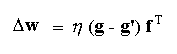## Assignment 3 -- The Delta Rule

#### Due by class time Wednesday, October 16

In Assignment 2, you should have observed that the linear associator quickly degrades as more and more information is stored.  A remarkably simple change to your program will allow you to implement a system that will correct errors, and which will sometimes converge to almost perfect answers even when a number of associations are to be learned.  This "teachable" and higher performance system will be useful by itself, and serves as the basis for a more complex learning algorithm for multi-layer networks called backpropagation.  Backpropagation is the most commonly used neural network algorithm.

The delta rule (or Widrow-Hoff or Adaline rule) updates the weights of the network based on the amount of error observed at the output layer.  The outer product form of the rule is shown below.Notice that if the matrix W is initially zero, g' will be zero and the learning rule is the same as the simple outer product Hebbian rule.  Also, if the entire set of inputs gives the correct associated outputs, so that (g - g') is always zero, then learning ceases.  This answers an important criticism of simple Hebbian systems where synapses are continually modified with learning and potentially can increase without bound.

The learning rate (eta) is usually a small constant between zero and one.  A trick sometimes used is to let eta be proportional to 1/n, where n corresponds to the nth learning trial.  Use of a decreasing learning rate is sometimes called tapering.  (Don't try tapering at first -- the system will work fine without using it, as long as you are not storing very many associations.)  If eta = 1 / fTf (i.e., the reciprocal of the square of the length of f), the system will respond perfectly immediately after adjusting the weights for the association f -> g.  (Do you see why this should be the case?)

Some care has to be taken with the value of eta.  If eta is too large, the system will oscillate and may diverge (try it and see).  Learning will overcorrect, and may actually increase the error.  If eta is too small the system will take a very long time to converge to a correct answer.  If eta is constant, W may oscillate around the "best" matrix, without ever converging to a final value.

Suppose we have a set of associations.  Suppose we present pairs from this set to the learning system at random.  If eta is relatively large (on the order of 1 / fTf), the association will be perfect (or nearly so) for the immediately past pair.  It will be less good if a pair has not been presented recently, because the corrections to later pairs may have corrupted the association between earlier pairs.  This error correction system displays some of the properties of a short term memory, in that it gives very good responses to the immedate past associations, and then gets progressively worse for more temporally distant associations.

#### Part 1

Your task for this part of the assignment is to repeat your studies of the linear associator from the last assignment using the delta rule learning procedure described above.

A good way to proceed is to keep a set of associations in a pair of arrays and then pick pairs at random, associating them using the error correction rule.  Add the resulting matrix of weight corrections to the developing weight matrix.  Keep going until done.  Part of the lore of error correcting models is that the pairs to be learned must be presented in random order.  Otherwise, strong sequential dependencies will be built into the matrix.  This behavior might be an interesting thing to investigate systematically.

Things to look for include:

• The oscillations mentioned above.
• How long it takes to converge.
• How many associations can be stored before the system starts to break down.
• What happens if the associations are presented in sequence rather than randomly.  (What happens in the original linear associator if we present associations to be learned in different sequences?)

#### Part 2

Now test your associator on some real-world data. The file cancer.in (also available on turing and pccs in /cs/cs152/data) contains actual clinical data for breast cancer presence based on 9 measured variables. This file follows a set format:

• The first number in the file is the dimensionality of the output patterns, say m.
• The second number in the file is the dimensionality of the input patterns, say n.
• The remainder of the file contains the data, with m output values followed by n input values. (The number of data patterns is not stated explicitly; your program should just read to the end-of-file.)
For the breast cancer data, m = 1 and n = 9. The single output value classifies the input pattern as either Benign (0) or Malignant (1). Each input pattern consists of 9 values between 0.0 and 1.0 representing the following variables:
• Clump Thickness
• Uniformity of Cell Size
• Uniformity of Cell Shape
• Single Epithelial Cell Size
• Bare Nuclei
• Bland Chromatin
• Normal Nucleoli
• Mitoses

Modify your program so that it can read in data patterns from a file. Then test your network by training it with the delta rule on some subset of the breast cancer data, until the error measure drops below some acceptable threshold. How well is it able to classify the training data? Next, test the network's generalization ability by testing it on patterns from the data set that were not included in the training data. Does it still produce correct classifications for these novel patterns? Experiment with different sizes of training/testing sets, different termination thresholds, and different learning rates.

Two smaller data files are also available for testing your network: nand.in and xor.in. How long does it take your network to converge on the nand patterns? What about xor?